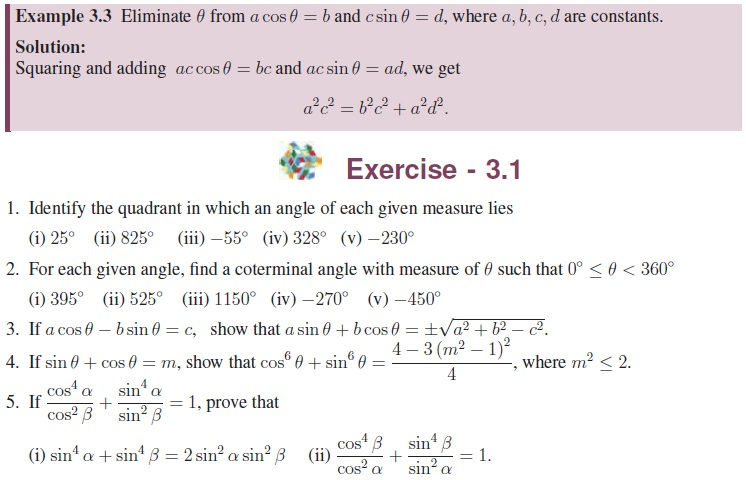Home | | Maths 11th std | A recall of basic results

# A recall of basic results

First, let us recall the definition of angle and degree measure of an angle.

A recall of basic results

In earlier classes, we have learnt trigonometric ratios using a right triangle and proved trigonometric identities for an acute angle. One wonders, how the distance between planets, heights of Mountains, distance between far off objects like Earth and Sun, heights of tall buildings, the speed of supersonic jets are measured or calculated. Interestingly, such distances or heights are calculated applying the trigonometric ratios which were derived for acute angles. Our aim is to develop trigonometric functions defined for any real number and use them in all branches of mathematics, in particular, in calculus. First, let us recall the definition of angle and degree measure of an angle.

## 1. Angles

The angle AOB is a measure formed by two rays OA and OB sharing the common point O as shown in the Figure 3.1. The common point O is called the vertex of the angle. If we rotate the ray OA about its vertex O and takes the position OB, then OA and OB respectively are called the initial side and the terminal side of the angle produced. An anticlockwise rotation generates a positive angle (angle with positive sign), while a clockwise rotation generates a negative angle (angle with negative sign).One full anticlockwise (or clockwise) rotation of OA back to itself is called one complete rotation or revolution.

## 2. Different Systems of measurement of angle

There are three different systems for measuring angles.

(i) Sexagesimal system

The Sexagesimal system is the most prevalent system of measurement where a right angle is divided into 90 equal parts called Degrees. Each degree is divided into 60 equal parts called Minutes, and each minute into 60 equal parts called Seconds.

The symbols 1ŌŚ”, 1 and 1 are used to denote a degree, a minute and a second respectively.

(ii) Centesimal system

In the Centesimal system , the right angle is divided into 100 equal parts, called Grades; each grade is subdivided into 100 Minutes, and each minute is subdivided into 100 Seconds. The symbol 1g is used to denote a grade.

(iii) Circular system

In the circular system , the radian measure of an angle is introduced using arc lengths in a circle of radius r. Circular system is used in all branches of Mathematics and in other applications in Science. The symbol 1c is used to denote 1 radian measure.

## 3. Degree Measure

The degree is a unit of measurement of angles and is represented by the symbol ŌŚ”. In degrees, we split up one complete rotation into 360 equal parts and each part is one degree, denoted by 1ŌŚ”. Thus, 1ŌŚ” is 1/360 of one complete rotation. To measure a fraction of an angle and also for accuracy of measurement of angles, minutes and seconds are introduced. One minute (1 ) corresponds to 1/60 of a degree and in turn a second (1 ) corresponds to 1/60 of a minute (or) 1/3600 of a degree.

We shall classify a pair of angles in the following way for better understanding and usages.

┬Ę        Two angles that have the exact same measure are called congruent angles.

┬Ę        Two angles that have their measures adding to 90ŌŚ” are called complementary angles.

┬Ę        Two angles that have their measures adding to 180ŌŚ” are called supplementary angles.

┬Ę        Two angles between 0ŌŚ” and 360ŌŚ” are conjugate if their sum equals 360ŌŚ”.## 4. Angles in Standard PositionAn angle is said to be in standard position if its vertex is at the origin and its initial side is along the positive x-axis. An angle is said to be in the first quadrant, if in the standard position, its terminal side falls in the first quadrant. Similarly, we can define for the other three quadrants. Angles in standard position having their terminal sides along the x-axis or y-axis are called quadrantal angles. Thus, 0ŌŚ”, 90ŌŚ”, 180ŌŚ”, 270ŌŚ” and 360ŌŚ” are quadrantal angles.

## 5. Coterminal angles

One complete rotation of a ray in the anticlockwise direc-tion results in an angle measuring of 360ŌŚ”. By continuing the anticlockwise rotation, angles larger than 360ŌŚ” can be produced. If we rotate in clockwise direction, negative angles are produced. Angles 57ŌŚ”, 417ŌŚ” and ŌłÆ303ŌŚ” have the same initial side and terminal side but with different amount of rotations, such angles are called coterminal angles. Thus, angles in standard position that have the same terminal sides are coterminal angles . Hence, if ╬▒ and are coterminal angles, then ╬▓ = ╬▒ + k(360ŌŚ”), k is an integer. The measurements of coterminal angles differ by an integral multiple of 360ŌŚ”.## 6. Basic Trigonometric ratios using a right triangle

We know that six ratios can be formed using the three lengths a, b, c of sides of a right triangle ABC. Interestingly, these ratios lead to the definitions of six basic trigonometric functions.

First, let us recall the trigonometric ratios which are defined with reference to a right triangle.## 7. Exact values of trigonometric functions of widely used angles## 8. Basic Trigonometric IdentitiesTags : Solved Example Problems, Exercise | Trigonometry | Mathematics , 11th Mathematics : UNIT 3 : Trigonometry
Study Material, Lecturing Notes, Assignment, Reference, Wiki description explanation, brief detail
11th Mathematics : UNIT 3 : Trigonometry : A recall of basic results | Solved Example Problems, Exercise | Trigonometry | Mathematics